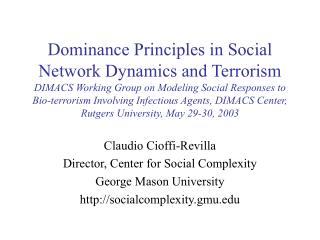DownloadDownload PresentationClaudio Cioffi-Revilla Director, Center for Social Complexity George Mason University socialcomplexity.gmu

# Claudio Cioffi-Revilla Director, Center for Social Complexity George Mason University socialcomplexity.gmu

Download Presentation## Claudio Cioffi-Revilla Director, Center for Social Complexity George Mason University socialcomplexity.gmu

- - - - - - - - - - - - - - - - - - - - - - - - - - - E N D - - - - - - - - - - - - - - - - - - - - - - - - - - -
##### Presentation Transcript

1. Dominance Principles in Social Network Dynamics and TerrorismDIMACS Working Group on Modeling Social Responses to Bio-terrorism Involving Infectious Agents, DIMACS Center, Rutgers University, May 29-30, 2003 Claudio Cioffi-Revilla Director, Center for Social Complexity George Mason University http://socialcomplexity.gmu.edu

2. Reference • Claudio Cioffi-Revilla (1998) Politics and Uncertainty: Theory, Models and Applications (Cambridge University Press), Part III (Micropolitics): Chapters 5-7. • Claudio Cioffi-Revilla & Harvey Starr (1995) “Opportunity, willigness and political uncertainty: theoretical foundations of politics,” Journal of Theoretical Politics 7:447-76.

3. Dominance Principles • A dominance principle is a formal theorem that states the relative sensitivity (elasticity) of the probability Pr(Y) = Y of a compound event Y to variation in (i) the number of elementary events N that cause Y and (ii) the component causal probabilities {pi}.

4. Compound events & terrorism • All interesting terrorism-related events are arguably compound events: • The success (or failure) of a warning system (Quarantelli) • The success of a terrorist strike (Shubik) • The detection of impending terrorist action (Kvach) • The success of a counterterrorism operation • The success of first-responders or mitigation measures (Sorensen) • Reaching any state in Glasser et al.’s model (CDC). Each arrow! • Key: each of these events, and others, is composed of more basic, elementary events that are causally linked by elementary conjunction and disjunction operations (often highly complex) defined on the complete set of causal events.

5. The structure function (Y) • Formally, each event occurrence Y is given by a structure function(Y) that specifies the causal conjunctions and disjunctions of the elementary events {X} that produce Y. • E.g., (Y) A [(B C)(D E)],  so Y occurs whenever A and either B and not C or D and E occur. • Remark: The s.f. (Y) is obtained through finite event analysis, by decomposing processes and parsing compound events (CC-R, 1998). • Thus, the probability of a compound event Y(pi) is derived from the corresponding s.f. (Y) associated with the causal occurrence of event Y. • For , the s.f. yields Y = a[(b - bc) + (d + e - de)]. 

6. MASON “M 3”: GeniPol)

7. MASON “4a”: war onset

8. Sensitivity sx(Y) • Let Y = ƒ(X). The sensitivity of Y (e.g. probability of a compound event) with respect to X (e.g., p or N) is defined by the relative first-order derivative (economists’ “elasticity”): (i) continuous case: sx(Y) = (∂Y/∂X)(X/Y) (ii) discrete case: sx(Y) = (YX + 1YX)(X/Y) • Remark: Sensitivities are always directly comparable because they are expressed in pure numbers (dimensionless).

9. First-order dominance • For first-order (serial) conjunction, if Y = p, where  is the number of necessary conditions, then p dominates . Formally, sp > s. • For first-order (parallel) disjunction, if Y = 1  (1 p), where  is the number of sufficient modes, then p dominates . Formally, sp > s. • Remark: In both cases variations in component probabilities p dominate variations in causal complexity ( or ) in terms of the effect on Y, so p is the preferred target of intervention (control variable) to increase or decrease Y assuming equal costs in percent changes.

10. So what? Applications • Given an anti-terrorist strategy based on  operations with individual probabilities p, is it more effective to increase p or to increase  (add redundancy)? • Given a terrorist attack based on  requisites each with probability p, is it easier to prevent attack by lowering p or by increasing ? • Given a response plan based on  requirements each with success probability p, is it better to decrease  or to increase p?

11. Nth-order dominance • Similar results can be derived for compound events of unlimited causal complexity, so long as the s.f. can be specified (modeled). • Examples: • second-order conjunctive-disjunctive compound events, causally based on AND (conjunction) with ORs (disjunctions) at each requirement • second-order disjunctive compound events, causally based on OR (disjunction) modes with ANDs (conjunctions) for each mode.

12. Extensions • Distribution models E.g., E(T) = (/)1 [1 + 1/( + 1)], for Weibull-distributed onset attacks. • Probability kinematics when p, ,  and other parameters vary over time • Vector fields (role of dynamics): Y  Ppp  PNn

13. Conclusions • The probability of compound events (arguably all terrorism events of interest) is affected in qualitatively and quantitatively different ways (differentiated impacts) by changes in the underlying causal probabilities and in their causal connections. • In general, changes in component probabilities have a greater effect on the overall probability of a compound event than do changes in the number of causal events (whether conjunctive or disjunctive). (“Causal uncertainty is more important than causal complexity.”) • Strategies to prevent, combat, and respond to terrorism must be cognizant of these differential sensitivities. • Intuitions and common perceptions about these phenomena are often misleading, erroneous, or outright dangerous.